Introduction to Sample size determination

In an experiment, experimenter is interested in the effect of certain process, intervention or change (treatment) on targeted objects (experimental units).

Sample size determination is to decide an appropriate sample size to achieve a desired probability that the clinical trial give statistically significant result,

which is known as the power of test.

Go to:

Procedures to identify the appropriate program to use

1.      Identify the aspects you want

There are 6 aspects:

Means, Proportions, Survival Analysis, Phase II Clinical Trial, Confidential Interval and Others

The test for correlation coefficient and standard normal calculator is provided in the “Others” category.

2.      For Means, Proportions,

i.                    Select the design you want: One sample design, Two samples parallel design or Two samples crossover design.

ii.                 Select the test you want: Equality, Non-Inferiority / Superiority, or Equivalence.

iii.               Please kindly follow the detailed procedures on the calculator to work out the sample size. Examples are also provided as reference.

iv.               For two proportions, besides choosing from designs,

You can also choose from Confidence Interval – Bristol and Compare Two Proportions – Casagrande, Pike & Smith

Note:

Please kindly find the detailed guidance and explanation of terms via the links.

3.

i.                    Select the comparison you want: One survival curve or two survival curves

ii.                 There are four choices:

1.      Comparison of Survival Curves Using Historical Controls

2.      Comparison of Two Survival Curves Allowing for Stratification

3.      Comparison of Two Survival Curves – Rubinstein

4.      Comparison of Two Survival Curves – Lachin

iii.               Please kindly follow the detailed procedures on the page to work out the sample size. Examples are also provided as reference.

The detailed calculation and formulae are shown in the Formula section on the calculator page.

Note:

For some terms of survival analysis appear on the calculator, please kindly find detailed explanation via the links.

To know more about survival analysis and the common terms, please click: Survival Analysis

4.      For Phase II Clinical Trials,

i.                    Select the technique you want:

ii.                 Please kindly follow the detailed procedures on the page to work out the sample size. Examples are also provided as reference.

Detailed explanation to the principle, choice of sample size, hypotheses, decision boundaries and

stopping criteria of the Phase II trial is shown in the ‘theory’ section on the calculator page.

Note:

For some terms of Phase II Clinical Trials on the calculator, please kindly find detailed explanation via the links.

To know more about Phase II Clinical Trials, please click: Phase II Clinical Trials

For the difference between Fleming’s method and Bayesian method, please click: Difference between Fleming’s and Bayesian method

5.      For Confidential Interval

i.                    Select the technique you want:

1.      One sample proportion

2.      Two sample proportions

3.      Correlation

4.      Single incidence rate

5.      Relative Risk and Attributable Risk

6.      Odds Ratios, ARR, RRR, NNT, PEER

7.      Diagnostic Statistics

8.      McNemar’s Test

ii        Please kindly follow the detailed procedures on the page to work out the sample size. Examples are also provided as reference.

Formulae and the definition of terms are shown on the page also.

6.      For Others,

i.                    Select the technique you want:

1.      Correlation Coefficient using z-transformation

2.      Standard Normal Calculator

ii.                 Please kindly follow the detailed procedures on the page to work out the sample size. Examples are also provided as reference.

Hypothesis Test

A statistical hypothesis test is a method of making decisions using data from a scientific study.

We have an original belief and now we have some evidence to suspect that the original belief is wrong and need to be updated.

Then we carry out hypothesis test to see whether the evidence is significant or not to disprove the original belief.

In statistics, a result is called statistically significant if it is unlikely to occur by chance alone

according to a pre-determined threshold probability called the significance level.

Definition of terms for hypothesis test and scientific experiment 

1.      Sample size (N)

The number of patients or experimental units required for the trial

2.      Treatment

The effect of certain process, intervention or change on objects

3.      Null hypothesis (H0)

A general or default position, i.e. no relationship between two treatments or a proposed medical treatment has no effect.

Experiment aims at rejecting the null hypothesis in a scientifically and statistically significant sense,

i.e. to prove the original belief to be false and update our conception. We reject the null hypothesis if it is not likely to occur.

4.      Alternative hypothesis (H1/Ha)

It is the alternative to the null hypothesis, suggesting there is relationship between two treatments in an unknown direction (two-sided)

or a specific direction (positive or negative, one side).

It is the position that our new evidence is suggested or is the position that we want to update our original belief.

An example of null hypothesis and two-sided alternative hypothesis is testing equality of means in one sample design:5.      Test statistic

It is a numerical summary or function of the observation, e.g. the mean of sample

In hypothesis test, we consider whether the value of observed test statistic is extreme by its distribution under the null hypothesis.

6.      Statistically significance

Statistical significance is a statistical assessment of whether observations reflect a meaningful pattern rather than a pattern by chance.

In statistics, a test statistic is believed to be statistically significant if it is more extreme than critical value, i.e. in the rejection region.Test Statistic

7.      Significance level (α)

It is a desired parameter of a cutoff probability in experimental design to determine whether an observed test statistic is extreme or not.

α is usually set to be 0.05, 0.025 or 0.01.

We reject the null hypothesis if the probability of the observed test statistic to appear is smaller than α.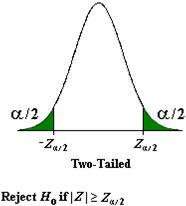8.      Critical value

It is the marginal value corresponding to a given significance level α.

This cutoff value determines the boundary that leads to the decision of rejecting or not the null hypothesis.

9.      p-value

It is the probability to obtain a new test statistic which is equal or more extreme than the original observed test statistic.

A small p-value indicates that it is unlikely to get the value of the observed test statistic.

We reject the null hypothesis if p-value is smaller than α.10.  Type I error

It is rejecting the null hypothesis when it is true, i.e. false positive.

α is the probability of type I error. It equals to the significance level in a simple null hypothesis.

11.  Type II error

It is not rejecting the null hypothesis when it is false, i.e. false negative.

Or say, not accepting the alternative hypothesis when it is true.

β is the probability of type II error. The power of test equals to 1-β12.  Power of test

The probability that a clinical trial will have a significant result, i.e. have a p-value less than the specified significance level α.

This probability is computed under the assumption that the treatment difference or strength of association equals the minimal detectable difference.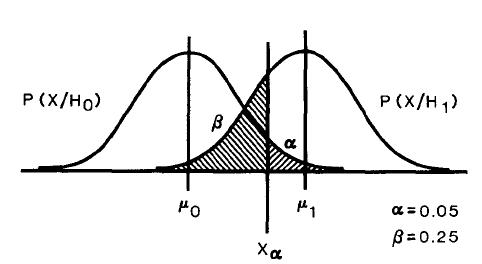The above figure shows the distribution of a test statistic X under null and alternative hypothesis.

As one increases sample size, the spread of the distributions in the above figure decreases, i.e.βdecreases (power increases).

Thus if the statistical test fails to reach significance, the power of the test becomes a critical factor in reaching an inference.

It is not widely appreciated that the failure to achieve statistical significance may often be related more to the low power

of the trial than to an actual lack of difference between the competing therapies. Clinical trials with inadequate sample size

are thus doomed to failure before they begin.

Thereforeone should take steps to ensure that the power of the clinical trial is sufficient to justify the effort involved.

The smallest difference between treatments you desire to be able to detect.

It is the smallest difference to be clinically important and biologically plausible in clinical trial.

14.  One-sided test

It is a test for particular direction, stated in the alternative hypothesis.

For example, it can be, choosing one of the directions in alternative hypothesis.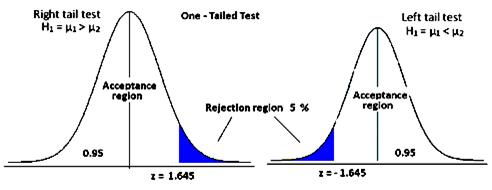15.  Two-sided test

It is a test for both directions, stated in the alternative hypothesis. For example,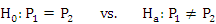E.g. One-sided test and two-sided test with same significance level = 0.05: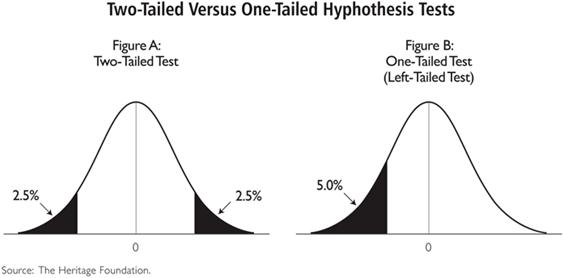Two types of experimental design

Parallel design

It is a design for a clinical trial in which a patient is assigned to receive only one of the study treatments.

It compares the results of a treatment on two separate groups of patients.

The experimental units (patients) are put into 2 groups randomly and each group receives one and only one treatment.

Then the results of treatment in two groups are compared.

Conducted properly, it provides assurance that any difference between treatments is in fact due to treatment effects (or random chance),

rather than some systematic differences between the groups of subjects.

For example, let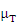and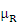be the mean of the response of the study endpoint of interest.

Also letandbe the inter-subject variance and intra-subject variance, respectively.

Assuming the equivalence limit is,, where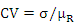and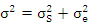(by Chow and Wang,2001)

Crossover design

It is a design for a clinical trial in which a patient is assigned to receive more than one of the study treatments.

It is a repeated measurements design such that each patient receives different treatments during the different time periods.

It compares the results of a set of treatments on the same group of experimental units (patients).

So in the design each patient serves as his/her own matched control.

The sequence of treatment received in each experimental unit is random.

For example, subject 1 first receives treatment A, then treatment B, then treatment C.

Subject 2 might receive treatment B, then treatment A, then treatment C.

It has the advantage of eliminating individual subject differences from the overall treatment effect, thus enhancing statistical power.

On the other hand, it is important in a crossover study that the underlying condition not changes over time,

and that the effects of one treatment disappear before the next is applied.

Therefore, it is usually use to study chronic disease and there is a wash-out period between each treatment to prevent carryover effect.

For example, define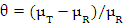and assume that the equivalence limit is, then, where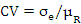(by Chow and Wang,2001)

Various types of test hypothesis 

1.      Testing equality

E.g.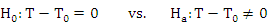Recall that the null hypothesis is a general or default position and is the position that we want to disprove or reject.

Alternative hypothesis is the position opposite to the null hypothesis and is the position that the new belief is suggested.

Hypothesis test check whether the evidence is significant or not to reject the null hypothesis (the original belief)

and establish a new belief (the alternative hypothesis).

It tests for the equality of a sample value with a targeted constant value or tests for the equality between treatment and active control/placebo.

Assume larger value indicates better performance. Null hypothesis states that the sample value equals the targeted value.

Alternative hypothesis is the sample value is not equal to the targeted value in either direction.

In two samples cases, testing equality is testing whether the values from 2 samples equal or not, i.e.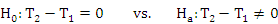2.      Testing non-inferiority/ superiority 

Non-inferiority: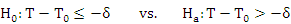Superiority: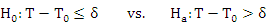Where δ>0 is the non-inferiority margin, or called superiority margin

Here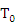represents the standard approved treatment/product; T represents the new treatment/product.

Or say,

 Test Null hypothesis Alternative hypothesis Non-inferiority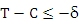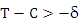Superiority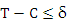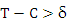Equivalence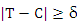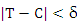WhereT: Treatment

C: Control

Assuming that the values to the right of zero correspond to a better response with the new drug

so that the values to the left indicate that the control is better,Non-inferiority means a treatment at least not appreciably worse than an active control/placebo by the non-inferiority margin δ.

That means the new treatment does not perform poorer than the active control/placebo appreciably.

This corresponds to the inequality suggested in the alternative hypothesis.

Conversely, inferiority means that a treatment is poorer than an active control/placebo by the non-inferiority margin δ.

Superiority means a treatment is more effective than the active control by the superiority margin δ, stated in the alternative hypothesis.

Conversely, non-superiority means that a treatment is not better than an active control/placebo by the superiority margin δ.

There are two types of superiority hypotheses, the above hypotheses are known as hypothesis for testing clinical superiority.

When δ=0, the above hypotheses are referred to as hypotheses for testing statistical superiority.

Q&A:

It may be confusing if you see this title the first time as when something, say “A”, is not inferior to “B”,

it means that “A” is not too worse than “B”, but not necessarily to be superior to (better than) “B” and vice versa.

Then how come we are testing for non-inferiority/ superiority.

Actually Testing non-inferiority/ superiority are two separate tests using the same setting of H0 and Ha, but with different signs in margin.

Assume that larger value of T represents better performance,

if the margin is -δ, then H0 means that test drug is inferior to the control. H1 is the non-inferiority of the test drug.

If the margin is δ, then H0 means test drug is not superior to control. H1 is the superiority of the test drug.

There is also confusion with the above Testing equality.

For Testing Equality, the equation corresponds to equality is stated in null hypothesis and it is what we want to reject.

Actually we expect to have difference between two treatments, as stated in the alternative hypothesis.

But by convention, we call this as testing equality.

Compared to Testing equality, in testing non-inferiority/ superiority, non-inferiority /superiority is stated in the alternative hypothesis.

The opposite, which is inferiority/non superiority of treatment and control, is stated in the null hypothesis.

That is we expect to the test drug to be superior/ not inferior to the control. We put what we expect in the alternative hypothesis.

E.g. In a test of superiority, to examine the effect of a test drug,

H0 is the response of test drug is less than that of placebo by δ.

Ha is the response of test drug is greater than that of placebo.

The test helps us to see whether the test drug is superior to the placebo by an amount of δ.

In two sample cases, testing non-inferiority/ superiority compares the values from two samples, i.e.

Non-inferiority: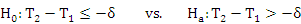Superiority: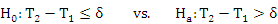Sample Size Determination 

For a superiority trial (S), the necessary sample size (N) depends on δs, the clinically important difference.

For a non-inferiority trial (NI), the necessary sample size depends on δNI, the upper bound for non-inferiority.

When δNI =δS, the necessary sample size for the non-inferiority trial is the same as superiority trial under the assumption of T-T0 = 0

On the other hand, δS is typically larger than δNI, which causes the sample size for a non-inferiority trial often to be much larger than that of a superiority trial.

3.      Testing equivalence

E.g.where δ > 0 is the margin of clinically accepted difference, called equivalence margin.

Here equality and equivalence are two different concepts.

Equality only focuses on whether the values are equal or not.

Equivalence means the difference of treatment and active control is within specific amount (δ) in either direction (positive or negative)

Note that the statement of equivalence is stated in the alternative hypothesis.

The inequality in the null hypothesis means that the treatment and control are not equivalence.

That means this test aims at proving the treatment and control are equivalence, therefore this new belief is put in the alternative hypothesis.

Null hypothesis states that the difference is at least δ.

Alternative hypothesis states that the difference is less than δ, i.e. equivalence.

In two sample cases, testing equivalence compares the values from two samples, i.e.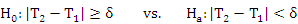Proportions Top

Confidence Interval – Bristol

It is used to determine the required sample size for a desired power of test and control the length of confidence intervals

of the difference of proportions not exceeding certain value,

compared with two samples parallel design test that only tests for the difference of proportions with a desired power.

The value of length is a bound to the length of confidential interval.

It is chosen relative to the expected length of the confidence interval, which is calculated by the formula on the webpage.

E.g. the expected length calculated is 0.141. Then you can find the sample size with the bound of length to be, say 0.2.

For binomial success probabilities, let π1 andπ2 denote the success probabilities of interest and let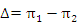Here are the large-sample normal approximations, as the exact results are very complicated

and the approximate results usually suffice for sample size determination.

For reference: 

First consider the problem of testing H0:Δ=0 against H1 :Δ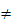0. Based on a sample of size n

from each distribution, let p1 and p2 denote the observed proportions of successes,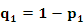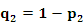,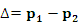With the hypothesis testing problem, Fleiss uses approximations based on the asymptotic normality

of the estimates to construct a confidence interval for A. The approximate (1 -α) 100 percent confidence interval forΔis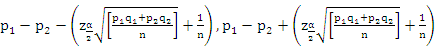(1)

and thus the associated hypothesis testing procedure is:

Rule: Reject Ho in favour of H1, if 0 is not an element of I, where I is the interval given in (1).

The length of the confidence interval given in (1) isOf course, this is a random variable and thus cannot be controlled. If the variance of the two normal distributions

described in the previous section had been unknown, then the length of the resulting confidence interval

for the difference of the means is based on the Student’s t-distribution and is also a random variable.

One approach to this problem is the determination of the expected length. The exact result is

difficult to obtain and is unnecessary for the problem of sample size determination.

An approximation to this expected length is: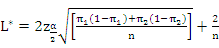Let nL denote the sample size required to have L*=L0, a specified positive value. It is straightforward to show that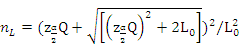where.

Note that, for fixedπ1, nL is maximized at, and symmetric about, π2 =0.5. Further, nL is symmetric in π1 and π2

and is maximized at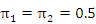Casagrande, Pike & Smith method 

It is a simple but accurate sample size approximation method for comparing two binomial probabilities.

It is shown that over fairly wide ranges of parameter values and ratios of sample sizes,

the percentage error which results from using the approximation is no greater than 1%.

You can choose one-sided or two-sided test in this method.

To find the minimum n to achieve a power of 100βpercent an iterative procedure is required.

This involves very extensive calculations and numerous approximations have thus been suggested.

The two most commonly employed are:

1.      The "arcsin formula" as given, for example, in Cochran and Cox (1957)

2.      The "uncorrected x2 formula" as given, for example, in Fleiss (1973)

Casagrande, Pike & Smith method is a Derivation of Corrected χ2

and is tested to be of good approximation over fairly wide ranges of values.

For details in calculation, please read “Casagrande, Pike and Smith (1978) Biometrics 34: 483-486

Survival Analysis

Survival analysis is the study of time between the entry into observation and the substantial events.

That means we observe the time needed until an event occur.

The events include death, relapse from remission, onset of a new disease and recovery.

It usually involves following the patients for a long time.

Some common terms in survival analysis: 

1.      Survival function [S(t)]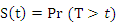where T is the time of failure or death

It is the chance that the subject survives longer than some specified time t.

2.      Hazard rate /Hazard function [λ(t)]

It is the instantaneous risk of occurrence of an event at time t, given the subject survives until time t or later.

Or it is the probability of failure in an infinitesimally small time period (t, t+Δt) conditional on survival until time t or later (that is, Tt)It is a risk measure. The higher the hazard function, the higher the chance of failure in the particular period.

Hazard function is a non-negative function. It can be increasing or decreasing.

3.      Hazard ratio (δ)

It is the ratio of the hazard rate in control group and hazard rate in experimental group.

It gives an instantaneous comparison between risk of failure in experimental group and control group.4.      Censoring

This refers to the value of an observation is only partly known. In survival analysis,

that is we have some information about the survival time of a subject, but do not know exactly when it fails.

It happen when a person does not encounter the event before the study ends, which is called “administratively censored”

or a person is lost to follow-up during the study.

5.      Prospective study

Prospective study is a study that follows over time a group of similar individuals who differ in certain factors under study

so as to determine the effect of these factors on the rate of outcome.

For survival analysis, the usual observation strategy is prospective study.

You start to observe in certain well-defined time point and the follow the patients for some substantial period

and finding out the time needed for an event to occur.

Note that the 4 methods below are not exact.

They describe the power function of the log-rank test only under the assumptions of a population model, but not distribution-free.

Thus, these methods have been proposed as approximations under the restrictive assumptions of proportional constant hazards,

i.e., under the exponential model. In reality, the hazards will not be constant, nor exactly proportional, over time.

The log-rank test will still be applicable but may not be maximally efficient.

Thus, it is our view that these methods should be cautiously applied using "worst-case" assumptions,

such as using the lowest plausible hazard rate for the control group, the smallest clinically relevant reduction in mortality,

and appropriate adjustments for departures from the usual assumptions of uniform patient recruitment, complete follow-up,

full compliance, and homogeneity over prognostic strata. But each model has certain generalization to release the assumption. 

Reference: Lachin and Foulkes (1986) Biometrics 42: 508

Prospective study and retrospective study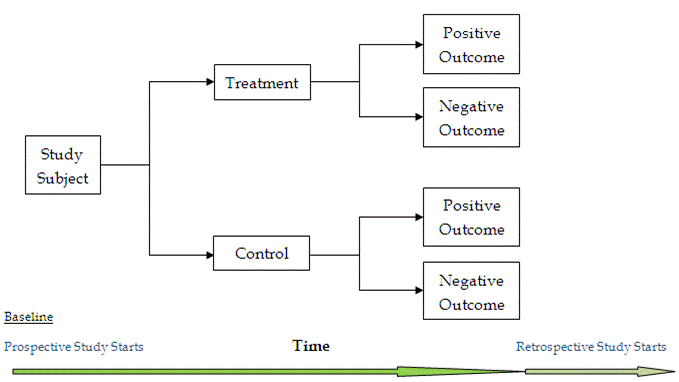Prospective

A prospective study watches for outcomes, such as the development of a disease,

during the study period and relates this to other factors such as suspected risk or protection factor(s).

The study usually involves taking a cohort of subjects and watching them over a long period.

The outcome of interest should be common; otherwise, the number of outcomes observed will be too small to be

statistically meaningful (indistinguishable from those that may have arisen by chance).

All efforts should be made to avoid sources of bias such as the loss of individuals to follow up during the study.

Prospective studies usually have fewer potential sources of bias and confounding than retrospective studies.

Retrospective

A retrospective study looks backwards and examines exposures to suspected risk or protection factors

in relation to an outcome that is established at the start of the study.

Most sources of error due to confounding and bias are more common in retrospective studies than in prospective studies.

For this reason, retrospective investigation is often criticized although it tends to be cheaper and faster than prospective study.

In addition, retrospective cohort studies are limited to outcomes and prognostic factors that have already been collected,

and may not be the factors that are important to answer the clinical question.

If the outcome of interest is uncommon and the required size of prospective study to estimate relative risk is often too large to be feasible,

the odds ratio in retrospective study provides an estimate of relative risk.

You should take special care to avoid sources of bias and confounding in retrospective studies.

Comparison of Survival Curves Using Historical Controls Top

It determines the number of patients needed in a prospective comparison of survival curves,

when the control group patients have already been followed for some period.

Explanation to variables:

α is the significance level for the test, usually 0.05

δ is the minimum hazard ratio, which is calculated by dividing the estimated hazard rate of control group by that of experimental group

MS is the median survival time in month in the control group, which can be estimated from existing control data.

r is the accrual rate. It is the rate of arrival of patients per month. It is estimated for future accrual.

nC and yC are the number of deaths observed and the number of patients still at risk in the historical control respectively.

Both are obtained from existing control data.

τ is the length of the planned continuation period for the study in months

T is the length of accrual period for the new study. It is the time needed to recruit patients into the trial.

Base on the accrual rate and the required number of accrual target and power of test,

you can find an appropriate accrual period to achieve desired power of test and required sample size.

So you adjust the variable T (accrual period) until desire power is obtained (e.g. 80%).

Several assumptions are made in this model. Firstly, It assumes time to survival is exponential distributed with hazard rate λ.

Secondly, It assumes prospective studies are used. It also assumes no withdrawal or losses to follow-up throughout the study.

The detailed calculation and formulae are shown in the Formula section on the calculator page.

Large randomized trials require longer time and higher cost, therefore the pilot investigations should be

carefully designed and analyzed. There are also diseases in which outcome is very predictable based on

known prognostic features and historically controlled studies may be viewed as an alternative to randomized clinical trials.

For some rare diseases, historically controlled study is suitable.

The accrual requirement declines as (i) the accrual rate declines,(ii) δ increases,

(iii) median survival in the controls decreases, (iv) the number of historical controls increases,

and (v) the number of failures already observed in the control group increases.

Reference: Dixon & Simon (1988) J Clin Epidemiol 41:1209-1213

Comparison of Two Survival Curves Allowing for Stratification Top

Stratification means patients are divided into homogeneous sub-groups called strata by a prognostic factor such as severity of disease.

Other properties can also be used such as Age > 50 or not, or male and female. In the calculator 2 strata can be set.

Explanation to variables:

α is the significance level for the test

β is the probability of type II error, or (1-power) of the test

K is the weight assigned to each stratum, identifying which one is more significant to the result.

It is usually proportional to sample size in each stratum.

δ is the minimum hazard ratio.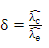It is calculated by dividing the estimated hazard rate of control group by that of experimental group

MS is the median survival time in month in the control group, which can be estimated from existing control data.

The sample fractions of control group (QC) and experimental group (QE) can be difference in each stratum and across two strata:T0 is the accrual period in month. It is the length of time to recruit patients for study in each stratum.

T-T0 is the follow-up period in month. It is the continuation period of all recruited patients to the end of study T in each stratum.

For detailed formula and theory, please check the Formula section on the calculator page.

Comparison of Two Survival Curves – Rubinstein

It is the determination of the number of patients needed in a prospective comparison of survival curves with losses to follow-up,

when the control group patients have already been followed for some period.

The explanation to variables is the same as above Allowing for Stratification” one.

Unlike the other model that only assume time to survival is exponential distributed with hazard rate λ, several assumptions are made under this model.

First, the arrival of patients is modeled by a Poisson process with rate n per year.

Then the patient is randomly assigned to the experimental group or control group, with equal probability each.

Second, the survival time for a patient is assumed to follow exponential distribution and is independent to each other.

Third, the time until loss to follow-up is assumed to follow exponential distribution and is also independent to each other.

The explanation to variables is the same as above Allowing for Stratification” one.

For detailed formula and theory, please check the Theory section on the calculator page.

Comparison of Two Survival Curves – Lachin

It determines the number of patients needed in a prospective comparison of survival curves,

when the control group patients have already been followed for some period.

It only assumes the survival time is exponential distributed with hazard rate λ.

In determination of sample size, it specifies the minimal relevant difference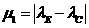The explanation to variables is the same as above Allowing for Stratification” one.

For detailed formula and theory, please check the Formula section on the calculator page.

Phase II clinical trials 

Phase II clinical trial typically investigates preliminary evidence of efficacy and continues to monitor safety.

There are three main objectives in treating patients in Phase II clinical trials.

The primary objective is to test whether the therapeutic intervention benefits the patient.

The second objective is to screen the experimental treatment for the response activity in a given type of cancer.

The final objective is to extend our knowledge of the toxicology and pharmacology of the treatment.

It involves usually fewer than 50 patients. Patients accrue in several stages in a multiple testing procedure,

testing being performed at each stage after appropriate patient accrual has been completed. The number of patients accumulates.

This feature is particularly appealing in a clinical setting where there are compelling ethical reasons to terminate a Phase II trial early

if the initial proportion of patients experiencing a tumor regression is too low or too high.

Phase II trials decide whether the new treatment is promising and warrants further investigation in a large-scale randomized Phase III clinical trial.

Phase II clinical trials are generally single-arm studies, but may take the form of multiple-arm trials.

Multiple-arm trials can be randomized or non-randomized with or without control arms.

It aims at estimating the activity of a new treatment.

These “pilot” studies are commonly applied to anticancer drugs to assess the therapeutic efficacy and toxicity of new treatment regimens.

Phase II clinical trials are only able to detect a large treatment improvement, e.g. greater than 10%.

To detect a small difference in treatment, e.g. less than 5%, one would require a much larger sample size,

which is not possible in Phase II studies due to the limited number of subjects eligible for the study

and the large number of treatments awaiting study.

Phase II studies are prominent in cancer therapeutics as new treatments frequently arise

from combinations of existing therapies or by varying dose or radiation schedules.

An important characteristic of some Phase II trial designs is the use of early stopping rules.

If there is sufficient evidence that one of the treatments under study has a positive treatment effect,

then patient accrual is terminated and this treatment is declared promising.

Also, if a treatment is sufficiently shown not to have a desirable effect,

then patient accrual is terminated and this treatment is declared not promising.

Difference between Fleming’s procedure and Bayesian design of Phase II clinical trials Top

This section describes both the hypotheses and design for Fleming’s and the Bayesian approach to single-arm Phase II clinical trials.

Both designs are used for Phase II clinical trials with binary outcomes and continuous monitoring.

The fundamental difference between the two designs is the frequentist basis for Fleming’s procedure

only depends on the observed results whereas the Bayesian approach uses prior information (Information from previous studies).

The testing procedure for Fleming’s procedure is based on the normal approximation to the

binomial distribution of the observed number of treatment responses.

The resulting decision boundaries, rg and ag, are solved analytically.

the observed results to yield revised beliefs about the treatment.

The testing procedure is based on the posterior probability of the experimental treatment given the observed data.

The posterior probability is a conditional probability computed from a beta distribution which results in the upper and lower decision boundaries,

Un and Ln, which are evaluated using numerical integration, namely “Simpson’s Composite Algorithm”.

Another difference between the two designs is that Fleming’s procedure has only two outcomes at the final recruitment stage, i.e. reject or accept H0,

while the Bayesian design traditionally allows for an inconclusive trial at the final stage (After attaining the maximum sample size set).

Simon’s Randomized Phase II DesignTop

In phase II clinical trial, randomized design is proposed to establish the sample size for the study

to obtain the treatment with greatest response rate for further / phase III clinical trial.

There are some advantages for randomized design:

1.      Randomization helps ensure that patients are centrally registered before treatment starts.

Establishment of a reliable mechanism to ensure patient registration prior to treatment is of fundamental importance for all clinical trials.

2.      Comparing to independent phase II studies, the differences in results obtained for the two agents will more likely represent

real differences in toxicity or antitumor effects rather than differences in patient selection, response evaluation, or other factors.

3.      In randomized phase II clinical trials, one is merely making a rational choice of one arm

and is free of any burden to prove statistically that the selected arm is superior.

Although it is desirable to select the best treatment,

selecting an arm that is equivalent to another or even slightly worse is not considered too grave a mistake.

Hence, the error rate to control is the probability of erroneously selecting an arm whose response rate is lower than

that of the best arm by an amount with medical importance (for example, 10%).

Similarly, the relevant power is the probability of correctly selecting an arm whose response rate is larger than

that of the second-best arm by an amount with medical importance (for example, 15%).

The formulae for the probability are shown on the calculator page.

Explanation to variables:

p is the Lowest response rate among all k treatments

k is the number of treatment arms

D is the difference in true response rates of the best and the next best treatment

Confidence Interval

Confidence interval (C.I.) is a range providing an interval estimate to true but unknown population parameter

and is used to indicate the reliability of an estimate.

It is an observed interval calculated from the particular sample, in principle different from sample to sample.

The confidence level (1-α) is the proportion of confidence intervals that cover the true parameter,

i.e. a 95% C.I. is the interval that you are 95% certain contains the unknown population true value.

Its relation with hypothesis test is that the 100(1-α)% confidence interval of the test statistic is the acceptance region of a 2-sided hypothesis test.

If the test statistic is more extreme than the upper or lower bound of the confidence interval, the null hypothesis is rejected.

The significance level of the test is the complement of the confidence level.

One sample proportion

Proportion is the number of success divided by the sample size. The calculator gives a confidential interval for the estimate.

Two sample proportions

It compares two proportions from independent samples and provides a confidential interval.

Confidence intervals of difference not containing 0 imply that there is a statistically significant difference between the population proportions.

Correlation

Correlation indicates whether two variables are associated.

It is a value from -1 to 1 with -1 representing perfectly negative correlation and 1 representing perfectly positive correlation.

The two variables should come from random samples and have a Normal distribution (or after transformation).

The confidence interval is a range which contains the true correlation with 100(1-α)% confidence.

Single incidence rate

Incidence rate is the rate at which new clinical events occur in a population.

It is the number of new events divided by the population at risk of an event in a specific time period, sometimes it is the person-time at risk.

Incidence is different from prevalence, which measures the total number of cases of disease in a population.

Thus, incidence carries information about the risk of having the disease, while prevalence indicates how widespread the disease is.

Relative Risk and Attributable Risk

 Disease No disease Totals Exposed a b n1=a+b Non-exposed c d n2=c+d Totals m1=a+c m2=b+d N=n1+n2

Relative Risk is the ratio of incidence of disease in Exposed group to that in Non-exposed group from a cohort/prospective study.

If Relative Risk is larger than 1, it is a positive association. If it is smaller than 1, it is a negative association.

Attributable Risk is the amount of disease incidence which can be attributed to an exposure in a prospective study.

Population Attributable Risk is the reduction in incidence if the whole population were unexposed, comparing with actual exposure pattern.

Relative Risk compares the risk of having a disease for not receiving a medical treatment against people receiving a treatment.

It can also compare the risk of having side effect in drug treatment against the people not receiving the treatment.

Attributable Risk and Population Attributable Risk tell the amount of risk prevented if we do not have certain exposure.

Exposed group is the group of patients exposed to certain factors of interest such as a new treatment, age 45 or above or smoking for 10 years or above.

Odds Ratios, ARR, RRR, NNT, PEER 

 Outcome Positive Outcome Negative Totals Feature Present a b n1=a+b Feature Absent c d n2=c+d Totals m1=a+c m2=b+d N=n1+n2

Odds Ratio (OR) refers to the ratio of the odds of the outcome in two groups in a retrospective study.

It is an estimate for the relative risk in a prospective study.

Absolute Risk Reduction (ARR) is the change in risk in the 2 groups and its inverse is the Number Needed to Treat (NNT).

Patient expected event rate (PEER) is the expected rate of events in a patient received no treatment or conventional treatment.

The Z-test for Odds Ratio shows whether the exposure affects the odds of outcome.

OR=1 means exposure has no effect on the odds of outcome.

OR>1 means exposure leads to higher odds of outcome and vice versa.

The Z-test for 2 Proportions shows whether there is difference between the proportions of events in 2 groups.

The Chi-square test for Association tests the association between the groups of feature and test result.

Diagnostic Statistics  Top

 Disease No disease Totals Test Outcome Positive a (True Positive) b (False Positive) n1=a+b Test Outcome Negative c (False Negative) d (True Negative) n2=c+d Totals m1=a+c m2=b+d N=n1+n2

Sensitivity is the ability of the test to pick up what it is testing for and Specificity is ability to reject what it is not testing for.

Likelihood ratios determine how the test result changes the probability of certain outcomes and events.

Pre-test and Post-test probabilities are the subjective probabilities of the presence of a clinical event or status before and after the diagnostic test.

For positive test, we find the positive post-test probability and for negative test, we find the negative post-test probability.

McNemar’s Test

 Test 2 Positive Test 2 Negative Totals Test 1 Positive a b n1=a+b Test 1 Negative c d n2=c+d Totals m1=a+c m2=b+d N=n1+n2

McNemar’s Test is a test on a 2x2 contingency table. It checks the marginal homogeneity of two dichotomous variables.

It is used for data of the two groups coming from the same participants, i.e. paired data

For example, it is used to analyze tests performed before and after treatment in a population.

Reference:

1.

2.

3.

4.

5.

6.

7.

8.

9.

10.

11.

12.

13.

14.

15.

16.

17.  Bristol (1989) Statistics in Med, 8:803-811

18.  Casagrande, Pike and Smith (1978) Biometrics 34: 483-486

19.  Lachin and Foulkes (1986) Biometrics 42: 507-519

20.  Dixon & Simon (1988) J Clin Epidemiol 41:1209-1213

21.  Lachin (1981) Controlled Clinical Trials 2: 94